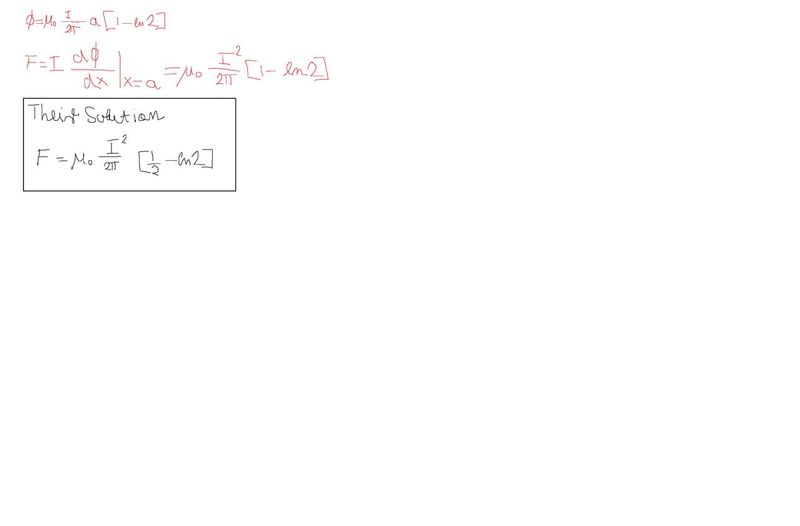# Magnetic force on the loop

## Homework Statement

Rigid contour of an isosceles triangle and a long line cable lie in a plane and are located in vacuum . Determine the intensity of the magnetic force F acting on the loop. Current I = 100 A.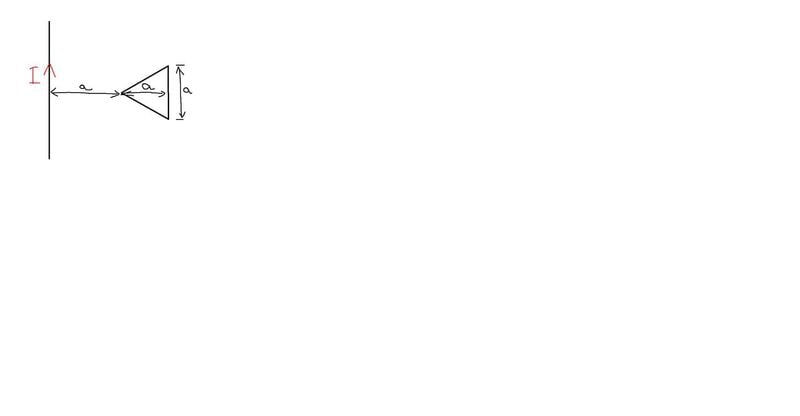F = 386 mN.
I am getting 2 mN.
Notice that I found dS by splitting while triangle in two triangles.

## The Attempt at a Solution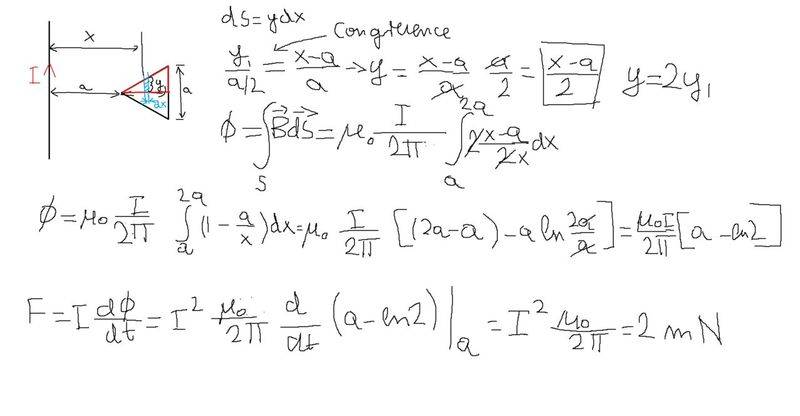Simon Bridge
Homework Helper
How is the derivative in the last line not zero?
Does the magnetic flux through the loop change with time?

In the problem description, the wire loop is not carrying a current.
ds is a line element and dS is a surface element... not always clear which you intend.
ie. The area element between x and x+dx, inside the triangle, is dS=2y(x)dx : y=(x-a)/2
So dS=(x-a)dx ... that what you mean?
Then: ##\vec B = \frac{\mu_0 I}{2\pi x}\hat k## and ##d\Phi = \vec B\cdot d\vec S##
... means the flux density $$\Phi = \frac{\mu_0 I}{2\pi}\int_a^{2a}\frac{x-a}{x}\text{d}x$$ ... which is where you ended up.

What about ##\vec F= I\oint (d\vec s\times \vec B)## ?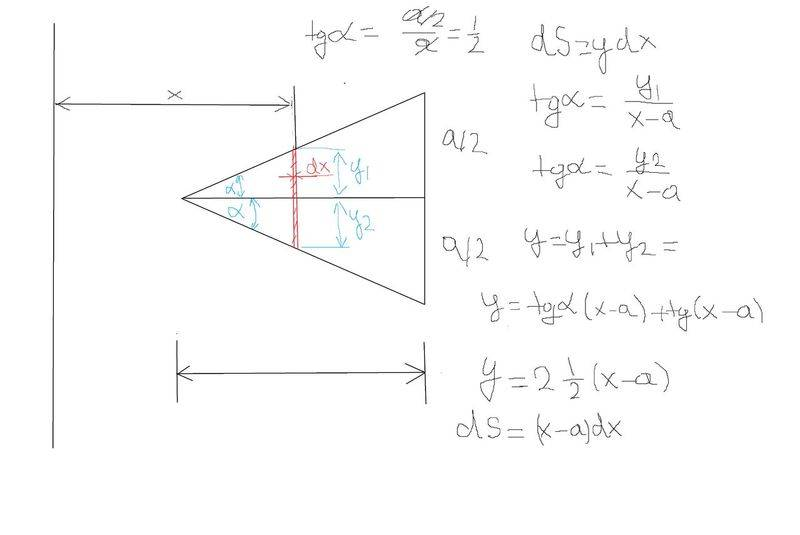You mean something like this , still I don't understand how there can be force on the loop if there is no current I flowing through loop.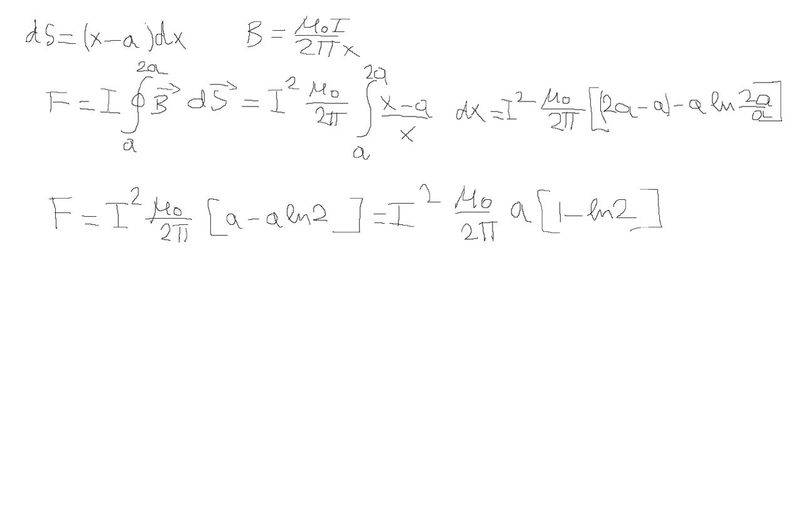image sharing

By the way I think I messed something up because a didn't cancle out in F.Guess because I didnt do the loop integral the right way

Last edited:
Please guys help me I am trying to figure out the whole day how my solution is incorrect, in the solution there is mi zero * I^2 / (2*pi) [1/2 - ln2] and I am having 1 - ln2 in the brackers , where did he get that one half from.